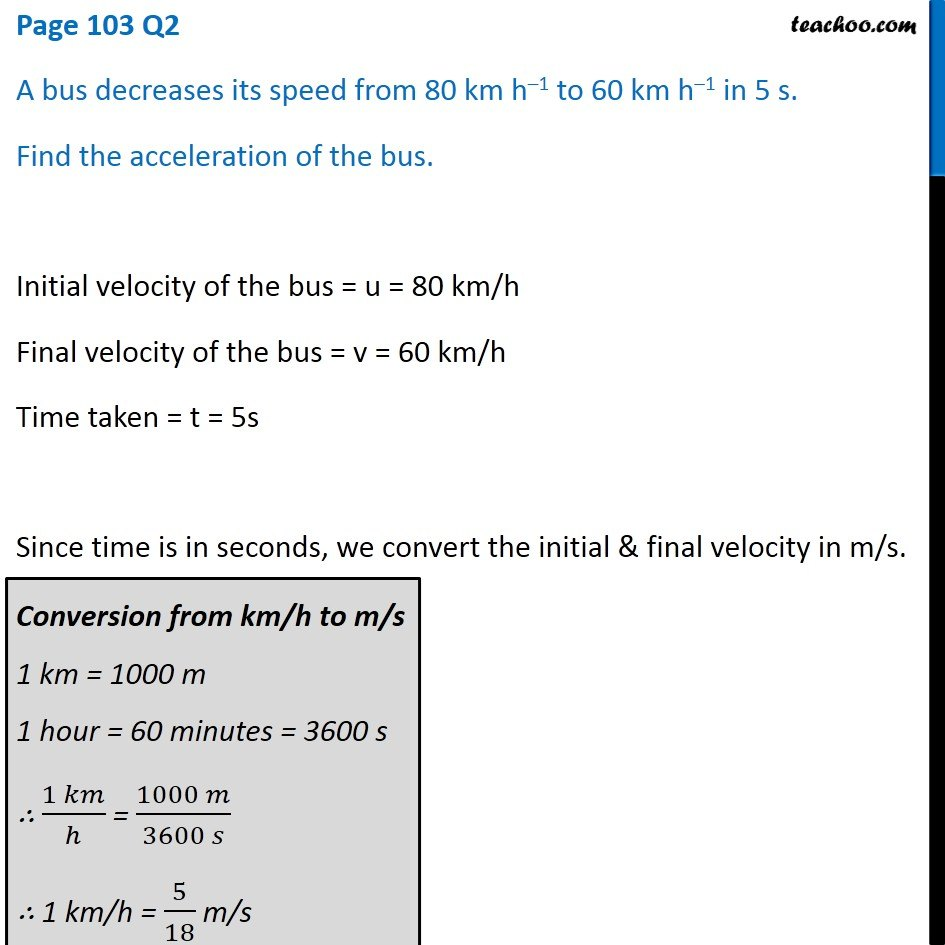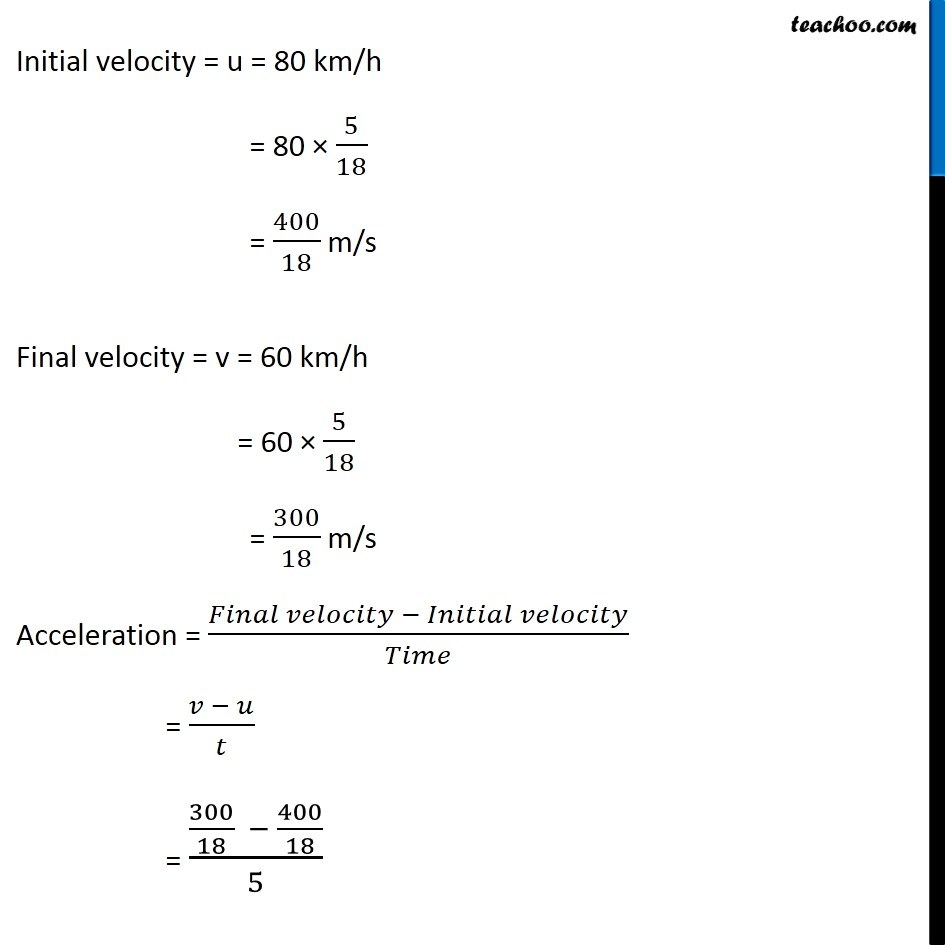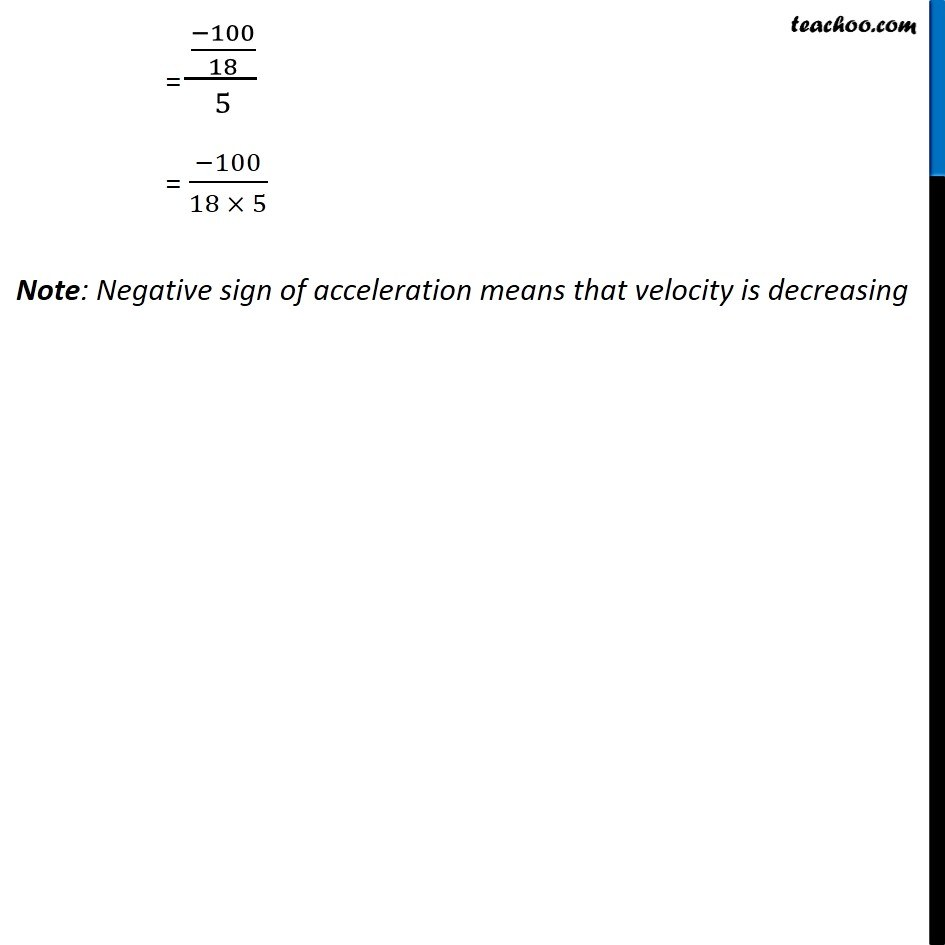Questions from Inside the chapter

Class 9
Chapter 8 Class 9 - MotionLearn in your speed, with individual attention - Teachoo Maths 1-on-1 Class

### Transcript

Page 103 Q2 A bus decreases its speed from 80 km h–1 to 60 km h–1 in 5 s. Find the acceleration of the bus. Initial velocity of the bus = u = 80 km/h Final velocity of the bus = v = 60 km/h Time taken = t = 5s Since time is in seconds, we convert the initial & final velocity in m/s. Conversion from km/h to m/s 1 km = 1000 m 1 hour = 60 minutes = 3600 s ∴ (1 𝑘𝑚)/ℎ = (1000 𝑚)/(3600 𝑠) ∴ 1 km/h = 5/18 m/s Initial velocity = u = 80 km/h = 80 × 5/18 = 400/18 m/s Final velocity = v = 60 km/h = 60 × 5/18 = 300/18 m/s Acceleration = (𝐹𝑖𝑛𝑎𝑙 𝑣𝑒𝑙𝑜𝑐𝑖𝑡𝑦 − 𝐼𝑛𝑖𝑡𝑖𝑎𝑙 𝑣𝑒𝑙𝑜𝑐𝑖𝑡𝑦)/𝑇𝑖𝑚𝑒 = (𝑣 − 𝑢)/𝑡 = (300/18 − 400/18)/5 = ((−100)/18)/5 = (−100)/(18 × 5) Note: Negative sign of acceleration means that velocity is decreasing